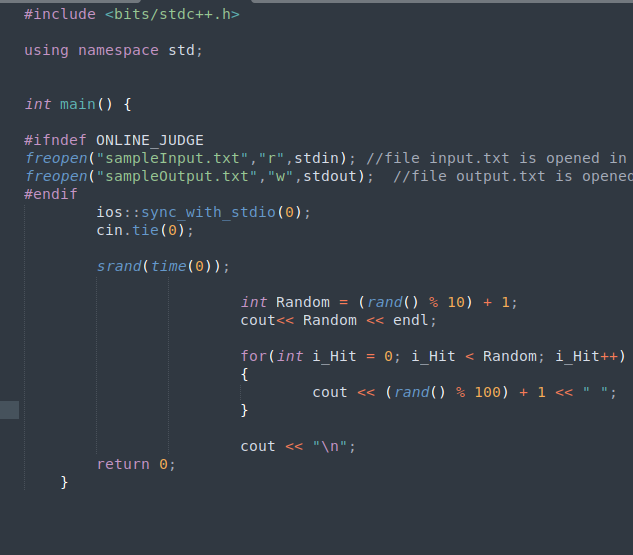Help Center

# Test case format

## This article contains information about test cases in programming questions and sample test cases for a sample programming question.

### Type of test cases required

Every programming question needs test cases. At HackerEarth, we recommend a minimum of 10 test cases that cover various corner cases for a specific problem statement. The format of the test case file should follow the given condition:

• There must be exactly 10 test cases for each problem.
• The total score of the test cases must be 100.
• Suppose the answer to any problem is a single integer or a single character, then it must have multiple test cases.
• The size of an individual test case should not exceed 8 MB.

These test cases can be divided as follows:

#### Easy test cases

Easy test cases should meet these conditions.

 Features of the test case Example Score Add two Easy test cases. Recommendation: The first two Easy test cases should be made visible to candidates If you have an array with size n equal to 10^5 constraints, then your n value should be less than equal to 10. Problem Constraint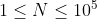Test Case Constraint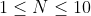If the total score of your question is 100, then your Easy test cases should have a score of 5 eachExample Test Case 1: 5 Marks Test Case 2: 5 Marks The constraint size should be minimal as possible The brute force approach can be used to solve the test case A candidate should be able to solve this test case manually

#### Medium test case

Medium test cases should meet these conditions.

 Features of the test case Example Score Add three Medium test cases If you have an array with size n equal to 10^5 constraints, then your n value should be less than equal to 10^3 Problem ConstraintTest Case Constraint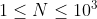If the total score of your question is 100, then your Medium test cases should have a score of 5 eachExample Test Case 3: 5 Marks Test Case 4: 5 Marks Test Case 5: 5 Marks These test cases should cover corner cases related to the algorithm being used A candidate should be able to solve these test cases in the most optimal manner. For example, a Greedy Algorithmic approach can be used to solve the test case.

#### Hard test cases

Hard test cases should meet these conditions.

 Features of the test case Example Score Add five Hard test cases If you have an array with size n equal to 10^5 constraints, then your n value should be less than equal to 10^5. Problem ConstraintTest Case ConstraintIf the total score of your question is 100, then your Hard test cases should have a score of 15 eachExample Test Case 6: 15 Marks Test Case 7: 15 Marks Test Case 8: 15 Marks Test Case 9: 15 Marks Test Case 10: 15 Marks These test cases are aimed at checking if candidates can solve the problem in the most optimal manner The constraint size should be as exhaustive as possible Only the correct algorithm or approach can be used to solve the test case

### Generating test cases

Generate test cases that are compatible with the auto-generated code snippets. For example, assume that you are creating test cases for this problem statement:

Write a program to find the highest/maximum number in an array.

#### Sample input and sample output

The input and output for this problem statement will be as follows: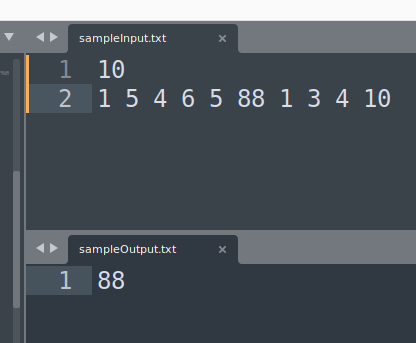### Explanation

• Here 10 in the first line of the sample input signifies the number of elements in the array
• The second line of the sample input signifies 10 elements in the array, separated by spaces
• The sample output only contains the answer that is the highest/maximum number in the array

So, go through the auto-generated code snippets first and then generate the test cases accordingly so that you don’t have to change the auto-generated code snippets afterward.

This is how you can easily create test cases for programming questions for various difficulties.

#### Solution for the example problem in C++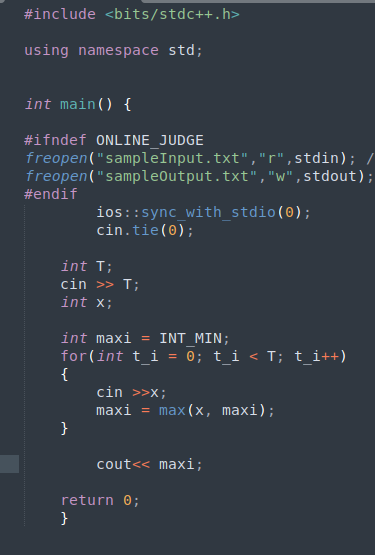### Code to create random test cases in C++ in your local system